Courses

# Magnetostatics

## 10 Questions MCQ Test Topicwise Question Bank for GATE Electrical Engineering | Magnetostatics

Description
This mock test of Magnetostatics for Electrical Engineering (EE) helps you for every Electrical Engineering (EE) entrance exam. This contains 10 Multiple Choice Questions for Electrical Engineering (EE) Magnetostatics (mcq) to study with solutions a complete question bank. The solved questions answers in this Magnetostatics quiz give you a good mix of easy questions and tough questions. Electrical Engineering (EE) students definitely take this Magnetostatics exercise for a better result in the exam. You can find other Magnetostatics extra questions, long questions & short questions for Electrical Engineering (EE) on EduRev as well by searching above.
QUESTION: 1

### The magnitude of H at a radius of 1 meter from along linear conductor is 1 A/m. The current in the wire is

Solution: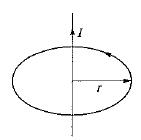Given, r = 1 m, H = 1 A/m
Using Ampere’s circuit law, we have: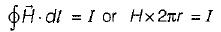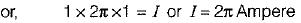QUESTION: 2

### What is the value of magnetic flux density at the centre of the square current loop shown in figure below?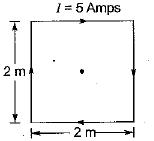Solution:

The magnetic flux density at the centre of the square having each side a is given by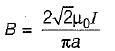Given, a = 2 m, I = 5 Amp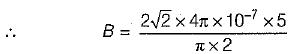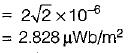QUESTION: 3

### A solenoid of 20 cm long and 1 cm diameter has a uniform winding of 1000 turns. If the solenoid is placed in a uniform field of 2 Wb/m2 flux density and a current of 10 amps, is passed through the solenoid winding, then the maximum torque on the solenoid will be

Solution:

Given, l = 20 cm = 0.2 m,
r - 0.5 cm = radius = 0.005 m
N = 1000,
B = 2 Wb/m2,
I = 10 A
The torque acting on the solenoid is
T = NIAB sinθ
T will be maximum when
θ = 90°, i.e. sin90° = 1
∴ Tmax = 1000 x 10 (0.005 x 0.2) x 2
= 20 N-m

QUESTION: 4

What is the value of magnetic flux density at the centre of a current carrying loop when the loop radius is 2 cm, loop current is 1 mA and the loop is placed in air?

Solution:

Magnetic flux density at the centre of a current carrying loop is given by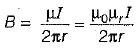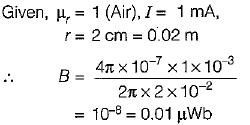QUESTION: 5

A magnetic field known to be directed in a cartesian co-ordinate system so that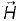exists in the x-direction where Hx = Constant. The value of curl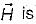Solution:

Curl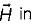cartesian coordinate is given by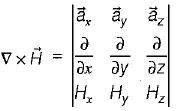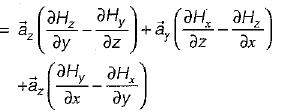Since Hx = constant and Hy = Hz = 0, therefore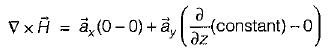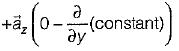= 0 + 0 + 0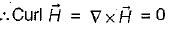QUESTION: 6

Match List-I with List-ll and select the correct answer using the codes given below the lists:
List-I
B. Maxwell equation in point form
C. Electric field strength general equation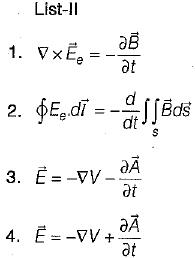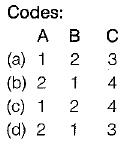Solution:
QUESTION: 7

The force acting between two parallel wires carrying currents I1 and I2 and separated by a distance r is given by

Solution:
QUESTION: 8

Flux lines are received at an iron-air boundary at an angle of 45° from the normal on the iron side of the boundary. If the iron has a relative permeability of 350, then the angle from the normal with which the flux emerges into the air would be

Solution: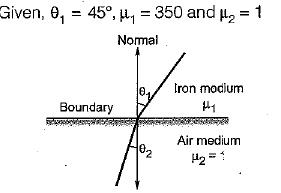Let medium-1 and medium-2 are respectively iron and air, then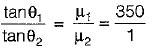or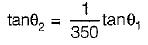or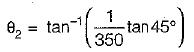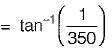QUESTION: 9

The unit of magnetic flux is given by

Solution:
QUESTION: 10

Assertion (A): The potential in case of magnetic field is a vector potential.
Reason (R): The potential in case of electric field is a scalar potential

Solution:
• Both assertion and reason are correct statements.
• The potential in case of magnetic field is a vector potential because source for producing a magnetic field is a current element which has both magnitude and direction.
• The potential in case of electric field i.e. electric potential is a scalar potential because the source for producing an electric field is a charge which is a scaler quantity (having only magnitude).